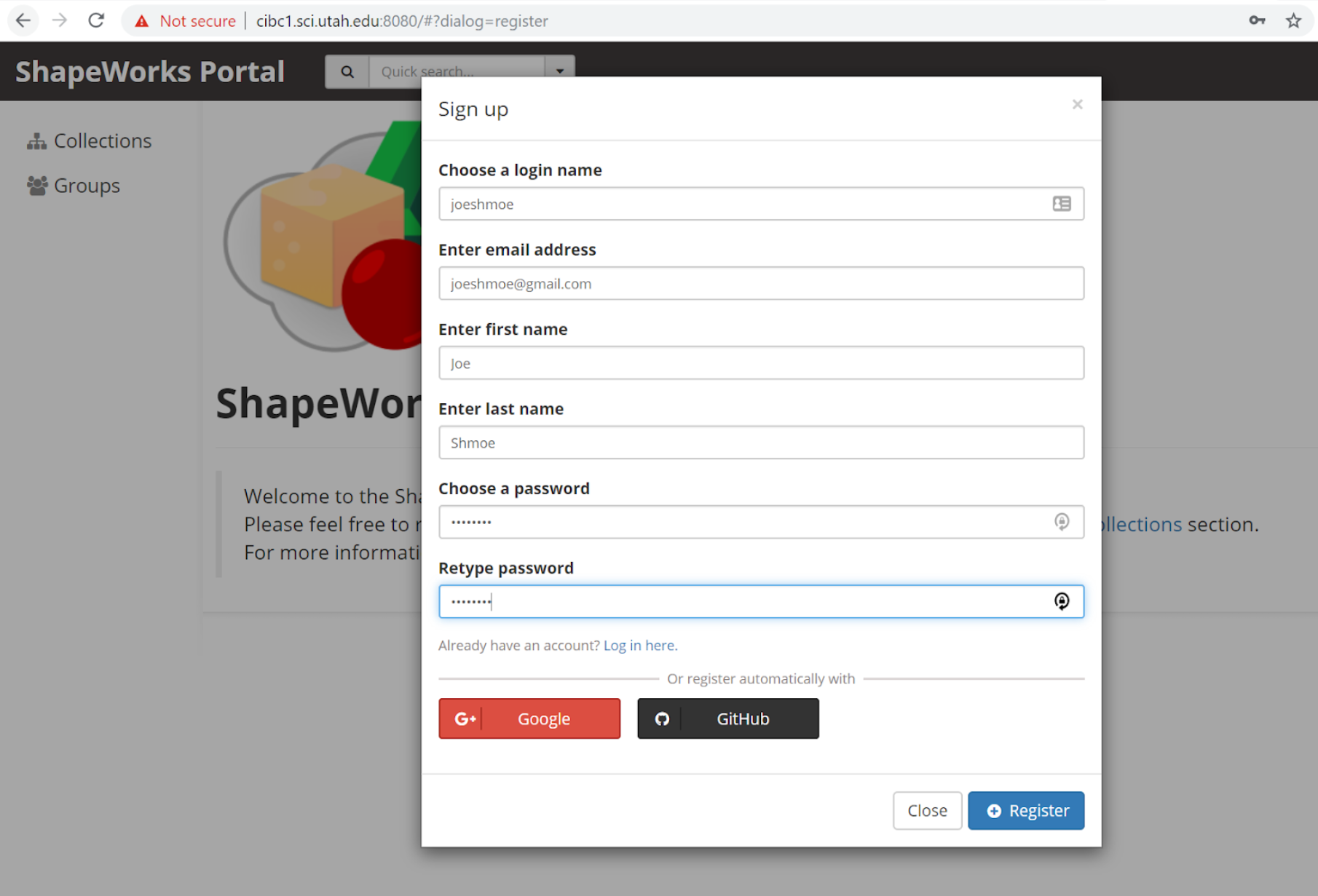# Use Cases

Use Case datasets such as the ellipsoid and left atrium datasets will be downloaded automatically from the ShapeWorks Data Portal. When running one of the use case example scripts, you will see something like this:

Can't find ellipsoid.zip in the current directory.
.___________________________.
|                           |
|     ShapeWorks Portal     |
|___________________________|

New ShapeWorks Portal users: Register an account at
http://cibc1.sci.utah.edu:8080/#?dialog=registerDanger

Do not use the same password as for your bank account or email!

Username: joeshmoe
[1/1 MB]


When a use case is run, it downloads the appropriate zipped data to Examples/Python/Data/. The data is then extracted to Examples/Python/output/use_case_name/ where all of the output from running the use case is also saved.

The downloaded data includes the raw data the use case starts with (segmentations, meshes, and/or images) as well as the output from the final grooming step of the use case (i.e. distance transforms) and the shape model generated from running the use case (particle files and xmls).

To visualize a shape model, open the included "analyze.xml" file can be opened in Studio. For example, to view the shape model downloaded for the ellipsoid use case run:

$cd Examples/Python/Output/ellipsoid/$ ShapeWorksStudio ellipsoid-v0/shape_models/ellipsoid/analyze_128.xml


## Running Use Case

The use cases are located at: Examples/Python/. When a use case is run, the dataset is automatically downloaded.

Important

You must first register of a free ShapeWorks account by visiting the ShapeWorks Data Portal.

To run a use case, run the following command from the Examples/Python/ directory:

$cd /path/to/shapeworks/Examples/Python$ python RunUseCase.py --use_case [insert name of use case here]


For names for the use cases that are currently released and the full list of optional tags, run:

$python RunUseCase.py --help  • --use_subsample: To run on a subset of the data in the use case, add the --use_subsample tag followed by the --num_subsample tag with the number of samples you wish to use. For example: $ python RunUseCase.py --use_case [insert name of use case here] --use_subsample --num_subsample 10


This will select a representative subset of the specified sample size to run through the pipeline so that the use case runs faster and uses less memory. The subset is selected by running clustering, then picking one sample from each cluster so that the resulting subset is representative of all the data. If --use_subsample is used without --num_subsample it will use the default number of subsamples which is 3.In order to run the clustering, the entire dataset will be downloaded.

• --interactive: When the interactive tag is used, the user must press enter after each step. This allows the user to inspect the intermediate output between steps of the pipeline.
$python RunUseCase.py --use_case [insert name of use case here] --interactive  • --skip_grooming: When this tag is used, the grooming steps are skipped. Instead of generating the distance transforms from segmentations via grooming, the distance transforms from the data .zip folder are used in optimization. If a user wishes to start with the optimization step, add --skip_grooming tag. $ python RunUseCase.py --use_case [insert name of use case here] --skip_grooming

• --groom_images: Some use cases can be run on just segmentations or on segmentations plus the corresponding imaging data. To carry the images through the grooming process with the segmentation, the --groom_images tag must be used. Note if this tag is used with a use case that does not have images, a warning will be printed and the tag will be ignored.
$python RunUseCase.py --use_case [insert name of use case here] --groom_images  • --use_single_scale: Use cases can be run with multi-scale or single-scale optimization. In both cases, particles on each shape sample are initialized using the particle splitting strategy starting from a single particle (or a given set of landmarks) until reaching the required number of particles. The optimized particles at each scale are used to initialize the next scale. At each scale, particles undergo initialization and optimization stages. The multi-scale triggers both the initialization and optimization stages, while the single-scale mode uses the initialization stage at each scale and runs the optimization stage when the required number of particles is reached (i.e., at the last scale). The default mode is mutli-scale. To run single-scale, use the --use_single_scale tag. $ python RunUseCase.py --use_case [insert name of use case here] --use_single_scale

• --tiny_test: Users can run a fast version of the use case by using the --tiny_test tag. This runs on a subset of the data for fewer optimization iterations to verify ShapeWorks has been properly installed. It is meant to test that use cases can run, not to create a good correspondence model.For tiny test,only a subset of the data will be downloaded directly in the Output folder and no zip file will be donwloaded in the Data folder.If the data has already been unzipped in Output folder, no new data will be downloaded.
$python RunUseCase.py --use_case [insert name of use case here] --tiny_test  • --shapeworks_path: This can be used to automatically add the ShapeWorks executable to the user's PATH variable if it has not already been set. To use this tag, add the --shapeworks_path tag followed by the path to the bin folder that contains ShapeWorks executables. $ python RunUseCase.py --use_case [insert name of use case here] shapeworks_path [path to shapeworks bin folder here]


## Running Subsequent Analysis

To run ShapeWorksStudio again without running the full pipeline, you must first navigate to the Examples/Python/ directory, and then run ShapeWorksStudio on the appropriate analysis XML file. For example:

$cd /path/to/shapeworks/Examples/Python$ ShapeWorksStudio TestEllipsoids/PointFiles/analyze.xml


## Dataset Guidelines

Check out How to Add New Datasets? for dataset upload instructions and guidelines.